# Conditional mathematical expectation

(diff) ← Older revision | Latest revision (diff) | Newer revision → (diff)

conditional expectation, of a random variable

A function of an elementary event that characterizes the random variable with respect to a certain-algebra. Let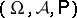be a probability space, letbe a real-valued random variable with finite expectation defined on this space and letbe a-algebra,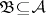. The conditional expectation ofwith respect to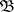is understood to be a random variable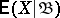, measurable with respect toand such that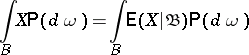(*)

for each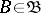. If the expectation ofis infinite (but defined), i.e. only one of the numbers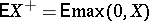and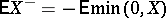is finite, then the definition of the conditional expectation by means of (*) still makes sense butmay assume infinite values.

The conditional expectation is uniquely defined up to equivalence. In contrast to the mathematical expectation, which is a number, the conditional expectation represents a function (a random variable).

The properties of the conditional expectation are similar to those of the expectation:

1)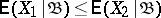if, almost certainly,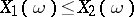;

2)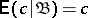for every real;

3)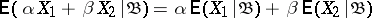for arbitrary realand;

4)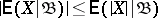;

5)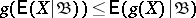for every convex function. Furthermore, the following properties specific to the conditional expectation hold:

6) Ifis the trivial-algebra, then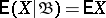;

7)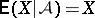;

8);

9) ifis independent of, then;

10) ifis measurable with respect to, then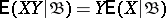.

There is a theorem on convergence under the integral sign of conditional mathematical expectation: If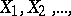is a sequence of random variables,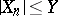,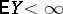andalmost certainly, then, almost certainly,.

The conditional expectation of a random variablewith respect to a random variableis defined as the conditional expectation ofrelative to the-algebra generated by.

A particular case of the conditional expectation is the conditional probability.

How to Cite This Entry:
Conditional mathematical expectation. Encyclopedia of Mathematics. URL: http://encyclopediaofmath.org/index.php?title=Conditional_mathematical_expectation&oldid=15801
This article was adapted from an original article by N.G. Ushakov (originator), which appeared in Encyclopedia of Mathematics - ISBN 1402006098. See original article